# Test: Design of Control Systems

## 20 Questions MCQ Test RRB JE for Electrical Engineering | Test: Design of Control Systems

Description
Attempt Test: Design of Control Systems | 20 questions in 60 minutes | Mock test for Electronics and Communication Engineering (ECE) preparation | Free important questions MCQ to study RRB JE for Electrical Engineering for Electronics and Communication Engineering (ECE) Exam | Download free PDF with solutions
QUESTION: 1

Solution:
QUESTION: 2

Solution:
QUESTION: 3

### The transfer function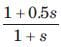represent a

Solution:
QUESTION: 4

A lag compensation network
(a) increases the gain of the original network without affecting stability.
(b) reduces the steady state error.
(c) reduces the speed of response
(d) permits the increase of gain of phase margin is acceptable.
In the above statements, which are correct

Solution:
QUESTION: 5

Phase lag controller:

Solution:

Phase lag controller is the integral controller that creates the phase lag and does not affect the value of the damping factor and that tries to reduce the steady state error.

QUESTION: 6

Consider the List I and List II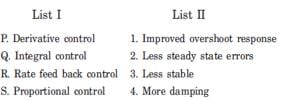The correct match is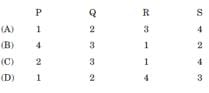Solution:
QUESTION: 7

A control system in which the control action is somehow dependent on the output is known as

Solution:
QUESTION: 8

The transfer function of a compensating network is of form (1 + αTs)/(1 + Ts). If this is a phase–Lag network, the value of α  should be

Solution:
QUESTION: 9

The poll–zero configuration of a phase–lead compensator is given by

Solution:
QUESTION: 10

While designing controller, the advantage of pole–zero cancellation is

Solution:
QUESTION: 11

Solution:
QUESTION: 12

The transfer function of a phase compensator is given by (1 + αTs) /(1 + Ts) where α > 1 and T >  0. The maximum phase shift provided by a such compensator is

Solution:
QUESTION: 13

For an electrically heated temperature controlled liquid heater, the best controller is

Solution:
QUESTION: 14

When phase-lag compensation is used in a system, gain crossover frequency, band width and undamped frequency are respectively :

Solution:
QUESTION: 15

A process with open–loop model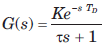is controlled by a PID controller. For this purpose
(A) the derivative mode improves transient performance
(B) the derivative mode improves steady state performance
(C) the integral mode improves transient performance
(D) the integral mode improves steady state performance.
The correct statements are

Solution:
QUESTION: 16

(a) improves response time
(b) stabilizes the system with low phase margin
(c) enables moderate increase in gain without affecting stability.
(d) increases resonant frequency
In the above statements, correct are

Solution:
QUESTION: 17

A Lag network for compensation normally consists of

Solution:
QUESTION: 18

The pole–zero plot given in fig. is that of a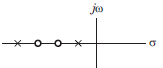Solution:
QUESTION: 19

The correct sequence of steps needed to improve system stability is

Solution:
QUESTION: 20

In a derivative error compensation

Solution:Use Code STAYHOME200 and get INR 200 additional OFF Use Coupon Code